• 声明：本篇博客根据回顾老师上课...创建哈希表时，把关键字为k的元素直接存入地址为H(k)的单元，以后查找关键字为k的元素时，再利用哈希函数计算该元素的存储位置。再按关键字存取元素。Hash中存储的key值都是唯一的。

声明：本篇博客根据回顾老师上课知识和书籍《数据结构——用C语言描述》（耿国华）整理得出，仅作知识回顾学习用。

1.哈希法

哈希法又称散列法、杂凑法、关键字地址计算法。相对应的表称为哈希表、散列表、或杂凑表等。

哈希方法思想
首先在元素的关键字k和元素的存储位置p之间建立一个对应关系H，使得 p = H(k)，H成为哈希函数。创建哈希表时，把关键字为k的元素直接存入地址为H(k)的单元，以后查找关键字为k的元素时，再利用哈希函数计算该元素的存储位置。再按关键字存取元素。Hash中存储的key值都是唯一的。
哈希冲突：
当关键字集合很大的时候，关键字值不同的元素可能会映射到哈希表的同一个地址，即k1 != k2，H(k1) == H(k2)，这种现象称为冲突，此时k1和k2为同义词。事实上冲突是不可避免的，由于关键字可能发生冲突的集合远远大于实际开辟的哈希表长度 ，构成冲突的必然性，可通过改进哈希的性能来减少冲突，即降低冲突的可能性。

2.哈希函数的构造方法

构造哈希函数原则
（1）函数本身便于计算
（2）计算出来的地址分布均匀，即对应任意一个关键字k，H(k)对应的不同地址的概率应该相同，以尽可能减少冲突

在实际应用中，构造哈希函数要考虑以下五个因素：
（1）计算哈希函数所需要的时间。哈希函数一定要简单，取放key值都需要根据哈希函数和key值计算位置，计算是要花时间的，尽可能要计算简单一点，这样计算时间也会少。
（2）关键字的长度。关键字过长，我们可以考虑取关键的某几位来建立哈希函数。
（3）哈希表的大小。哈希表可以减少查找次数，但是哈希表过短，或者过长都会使哈希法性能降低。
（4）关键字分布情况。为了使key值和哈希函数计算出来的地址分布均匀，要考虑关键字分布情况建立合适哈希函数。
（5）记录查找的频率。

2.1数字分析法

方法
首先大概了解关键字集合。如果每个关键字的位数比哈希表的地址码位数多时，可以从key值选择分布均匀的若干位，构成哈希地址。
比如哈希表长度为100，即地址空间为0~99，如果有200个key值，且key值都是8位十进制数d1d2d3d4d5d6d7d8，那么我们可以选择分布较均匀的两位数字作为key值。比如说所有key中，第二位和第五位数字都分布较均匀（即200个key值的第二位第五位都比较随机），那么我们就取d2d5作为key值地址码，比如 34982748，地址码为42。
（在这里存在不相同的key值，但是地址码一样，这是哈希冲突，哈希冲突解决办法在最后给出。哈希表，一个地址码可以代表（存放）一组数据）。

2.2平方取中法

方法
当无法确定关键字中哪几位分布较均匀时，可以求出关键字的平方值，再结合哈希表长度，取平方值的中间几位作为哈希地址（比如哈希表长度是 100，那哈希地址就00-99，我们就取key值平方后的中间2位作为地址码；如果哈希表长度是1000，那么哈希地址可以是000-999，那么就取key值平方后的中间3位作为地址码）。这是因为完成平方运算后，中间几位和关键字的每一位都相关，所以不同关键字会以较高的概率产生不同的哈希地址。

2.3分段叠加法

按哈希表地址位数将关键字分成位数相等的几部分（最后一部分可以较短），然后将这些部分相加，舍弃最高进位，剩下的数字作为地址码。比如哈希表长度为1000，可以表示地址码000-999。我们就把key值分成三位数为一部分。具体折叠方法有移位法和折叠法。以为叠加法直接将每一部分相加。折叠叠加法是将奇数段和偶数段分开，奇数段（偶数段）正常，偶数段（奇数段）倒序，再相加。

举例：12360324711202065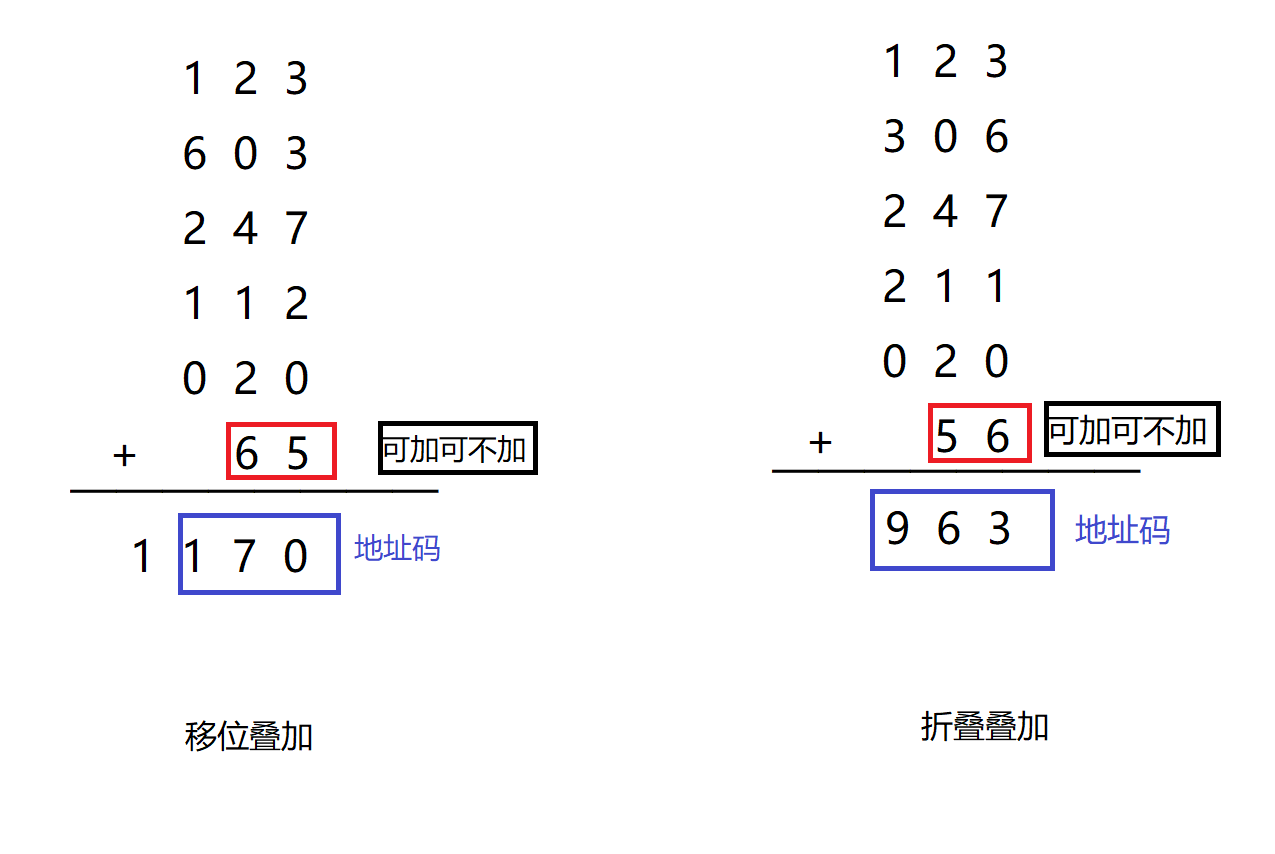2.4除留余数法

顾名思义，即对key值进行取余。看有多少key值，如果有几十个key值，我们可以选择对10取余，对15取余，等等。余数就是对应的地址码。

其实不论什么方法，目的都是为了在保证哈希函数尽可能简单的情况下让key值计算出来的地址码尽可能均匀。即让哈希表起到提高查找效率的作用。所以不管什么方法，只要达到最终这个目的就行。

2.5伪随机数法

即用一个为随机函数作为哈希函数p = H(key) = random(key)。

3.处理冲突的方法

3.1开放定址法

也叫做再散列法
思想：当关键字key的初始哈希地址p0 = H(key)出现冲突时（即该地址有key值了），以p0为基础，产生另一个地址p1，如果p1仍然冲突，再以p0为基础，产生另一个哈希地址p2…直至找到一个不冲突的地址pi，将key值存到里面。
即hi = (h0 + di)%m
m为表长，m为增量序列
（1）线性探测再散列
di = 1,2,3,4,…,m-1
（2）二次探测再散列
di = 1^2, -1^2, 2^2, -2^2,…, k^2, -k^2(k <= m/2)

3.2再哈希法

同时构造多个不同的哈希函数：
Hi = RHi(key)
哈希地址H1 = RH1(key)差生冲突时，再计算H2 = RH2(key)…直到冲突不再产生。

3.3建立公共溢出区

哈希表分为基本表溢出表两部分，凡是和基本表发生冲突的元素一律填入溢出表。

3.4链地址法

哈希表里每个表格是一个地址码和一个指针。将对应的key值都放在（key,指针）这种空间中，连接在对应的地址码后面。比如我们哈希表的地址码是key值对10取余。
有数10，12 ，32，34，92，33，11，67，那么都映射到哈希表上，对应的结构为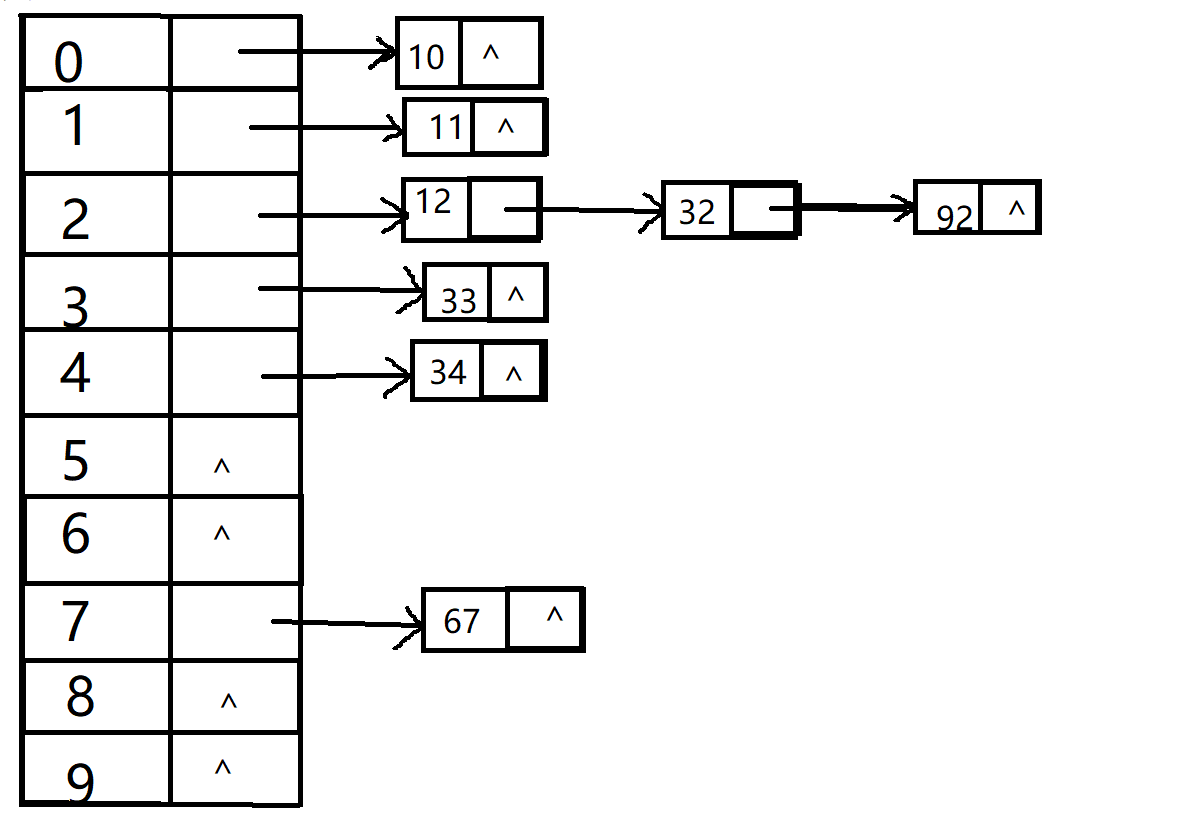结构体类型如下：

#define TABLESIZE 10
typedef int KeyType; // 键值的类型
typedef struct
{
KeyType key; // 学生信息 学号
//....其他数据成员 姓名
//....其他数据成员
}DataType; // 存储的数据类型

typedef struct
{
DataType key;
KeyNode* next;
}KeyNode;//key以这种结点方式被连接

typedef struct Node
{
KeyNode* next;//指向KeyNode类型的结点
}List;//哈希表每个格子的结构

typedef struct Hash
{
List* hash_table[TABLESIZE];
}ListHash;//哈希表

展开全文数据结构
• 1012: 哈希表（链地址法处理冲突） 题目描述 采用除留余数法（H（key）=key %n）建立长度为n的哈希表，处理冲突用链地址法。建立链表的时候采用尾插法。 输入 第一行为哈西表的长度m； 第二行为关键字的个数n； 第三...

1012: 哈希表（链地址法处理冲突）

题目描述
采用除留余数法（H（key）=key %n）建立长度为n的哈希表，处理冲突用链地址法。建立链表的时候采用尾插法。
输入
第一行为哈西表的长度m；
第二行为关键字的个数n；
第三行为关键字集合；
第四行为要查找的数据。
输出
如果查找成功，输出该关键字所在哈希表中的地址和比较次数；如果查找不成功，输出-1。
样例输入
13
13
16 74 60 43 54 90 46 31 29 88 77 78 79
16
样例输出
3,1

思路：
哈希表（以下内容重在理解）

1. 哈希表的基本概念： 哈希表（Hash table，也叫散列表），是根据关键码值(Key value)而直接进行访问的数据结构。也就是说，它通过把关键码值映射到表中一个位置来访问记录，以加快查找的速度。这个映射函数叫做散列函数，存放记录的数组叫做散列表。
• （tips:我对哈希表的浅显理解是将他比作图书馆书架，我们以后处理的数据的量少则十几万多则上亿，这个时候就用类似图书馆里的分类方法，分类后将数据存放在我们的电脑里，这样就会使得数据的查找较为简单。）
1. 哈希表的构建方法
① 直接定址法:（了解就好）
是以关键字 k 本身或关键字加上某个常量c 作为哈希地址的方法。直接定 址法的哈希函数 h(k)为: h(h)=k+c 例如,图 9. 30 所示的哈希表就是采用了这种方法。 这种方法的特点是哈希函数计算简单。当关键字的分布基本连续时， 可用直接定址法的哈希函数；否则,若关键字的分布不连续将造成内存单 视频讲解 元的大量浪费。

②除留取余法：（重点）
是用关键字k除以某个不大于哈希表长度m的整数p所得的余数作为哈 希地址。除留余数法的哈希函数 h(k)通常为： h(h)= k mod p（mod 为求余运算,p≤m) 除留余数法的计算比较简单,适用范围广,是最经常使用的一种哈希函数。这种方法的 关键是选好 p,使得元素集合中的每一个关键字通过该函数转换后映射到哈希表范围内的 任意地址上的概率相等,从而尽可能减少发生冲突的可能性。例如,p 取奇数就比取偶数 好。理论研究表明,p 取不大于m的素数时效果最好。

③数字分析法：（了解就好）
该方法是提取关键字中取值较均匀的数字作为哈希地址，它适用于所有关键字值都已知的情况，并需要对关键字中每一位的分布情况进行分析。

2. 哈希表的冲突解决
①开放地址法

• 就像某个人买了一张电影票，他晚到了电影院,他的位置被其他人占了,他就在周围找一个空座位坐下来。那么怎么找空闲单元呢？据开放定址法找空闲单元的方式又分为线性探测法和平方探测法等。

a.线性探测法
是从发生冲突的地址(设为 d)开始,依次探测 d,的下一个地 址(当到达下标为m-1的哈希表表尾时,下一个探测地址是表首地址 O),直到找到一个空闲 单元为止(当m>n时一定能够找到一个空闲单元)。

• 以前面的看电影为例,假设 电影院座位只有一排(共 20 个座位),他的座位是 8(被其他人占了),线性探测法就是依次 查看 9.10.…,20 的座位是否为空的,有空就坐下,否则再查看 1,2,…,7 的座位是否为空 的,如此这样,他总可以找到一个空座位坐下。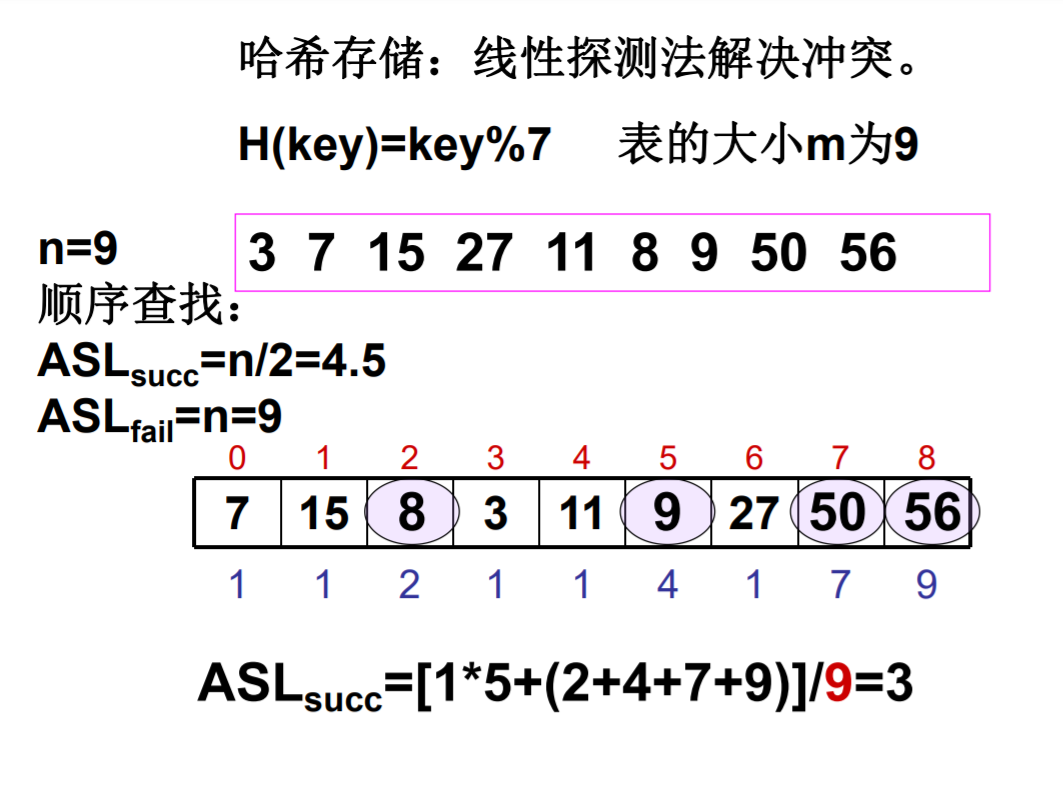b.平方探测法
设发生冲突的地址为 d,平方探测法(square probing)的探测序列为 d+1, d一1, d十2^1 , d一2^1,…。平方探测法的数学描述公式为： d,= h(k) d,=(d,±i) mod m (1≤i≤m-1)

• 仍以前面的看电影为例,平方探测法就是在他被占用的座位前后来回找空座位。 前后一个没找到就前后两个，找到为止。

②拉链法：
把具有相同散列地址的关键字(同义词)值放在同一个单链表中，称为同义词链表。有m个散列地址就有m个链表，同时用指针数组T[0…m-1]存放各个链表的头指针，凡是散列地址为i的记录都以结点方式插入到以T[i]为指针的单链表中。T中各分量的初值应为空指针。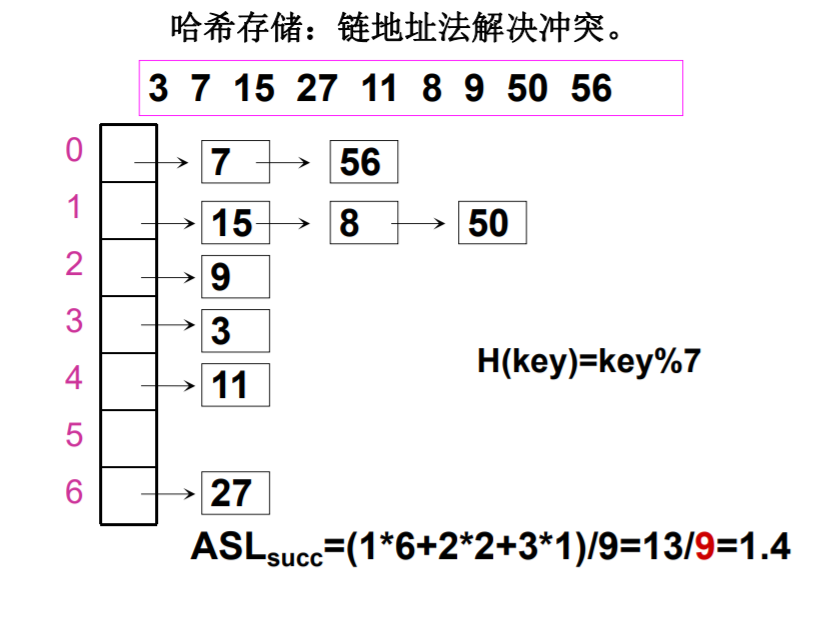• 看似复杂的拉链法，其实就是用一个“数组”把几个链表串起来罢了。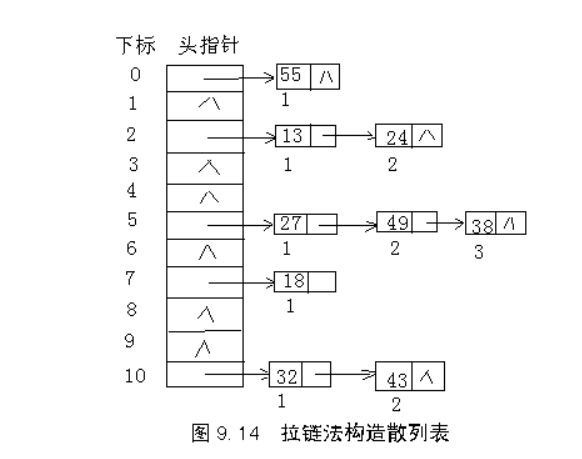【例】原本应该放在a的数据a发现a被占了，他就在a接一个链表成为a的“小弟”，后面相同类型的数据a1也是如此，他发现a被占了，还发现的a的“第一小弟”被占了，那就在“第一小弟数据a”后面接一个链表成为“a的第二小弟”，注：xxxx为空。1. 【拓展】哈希表的优缺点

优点：
无论数据有多少，处理起来都特别的快
能够快速地进行 插入修改元素 、删除元素 、查找元素 等操作
代码简单（其实只需要把哈希函数写好，之后的代码就很简单了）
缺点：
哈希表中的数据是没有顺序的
数据不允许重复

法一、拉链法
采用除留余数法（H（key）=key %n）建立长度为n的哈希表，处理冲突用链地址法。建立链表的时候采用尾插法。

#include<bits/stdc++.h>
using namespace std;
struct HTNode//储存值和下一个结点的结构体
{
int key;
struct HTNode *next;
};
struct HashTable//储存头结点的结构体
{
};
void InstHT(HashTable ha[],int n)
{
for(int i=0;i<n;i++)
{
}
}
void CreateHT(HashTable ha[],int n,int data)//创建哈希表
{
int t=data%n;//计算哈希地址序号
{
HTNode *p;
p=(struct HTNode*)malloc(sizeof(struct HTNode));//p=new HTNode ();
p->key=data;//储存数据
p->next=NULL;//将下一个结点设置为空
}
else//如果头结点不为空，则从头结点一直往后找到最后一个结点
{
HTNode *p,*q;
while(p->next!=NULL) p=p->next;//查找到最后一个结点
q=(struct HTNode*)malloc(sizeof(struct HTNode));//q=new();
q->key=data;
q->next=NULL;
p->next=q;//将新的结点接到最后
}
}
void SearchHT(HashTable ha[],int n,int data)//查找哈希表
{
int t=data%n;//计算哈希地址序号
HTNode *p;
for(int i=0; ;i++)//查找对应序号的链表
{
if(p==NULL)//如果为空，则没有，输出-1
{
printf("-1");//cout<<"-1";
break;
}
else if(p->key==data)//如果有，则输出答案，并且跳出循环
{
printf("%d,%d",t,i+1);//cout<<t<<i+1;
break;
}
else p=p->next;//否则继续向后查找
}
}
int main()
{
int n,k,data;
scanf("%d",&n);//cin>>n;
scanf("%d",&k);//cin>>k;
HashTable ha[n];
InstHT(ha,n);
for(int i=0;i<k;i++)
{
scanf("%d",&data);//cin>>data;
CreateHT(ha,n,data);
}
scanf("%d",&data);//cin>>data;
SearchHT(ha,n,data);
}

（代码出自孤雪胜悲鸣

法二、奇淫技巧之二维数组

#include<bits/stdc++.h>
using namespace std;
int main()
{
int i,j=0,a={0},m,n,key,b={0};    //m为哈希表长度，n为关键字的个数
scanf("%d %d",&m,&n);//cin >> m >>n;
for(i=0;i<n;i++)
{
scanf("%d",&key);//cin>>key;
a[key%m][b[key%m]++]=key;               //将同一类型关键字放入同一行。
}
scanf("%d",&key);//cin>>key;
for(i=0;i<n;i++)
{
if(a[key%m][i]==key){
printf("%d,%d",key%m,i+1);  //cout << key%m <<i+1;                   //查找对应的关键字时直接遍历该行数，并输出在二维数组中所在位置与查找次数
j++;
break;
}
}
if(j==0) printf("-1"); //cout << "-1";                          //如果没有找到，则输出-1
return 0;
}

以上方法仅供参考，欢迎互联网的广大朋友们提出指正。

展开全文链表 数据结构 哈希表 c++ c语言
• 哈希表（哈希查找） ...文章目录哈希表（哈希查找）哈希表概念哈希函数构造方法直接定址法数字分析法平方中法折叠法除留余数法处理冲突开放定址法线性探测二次探测再哈希法链地址法性能分析 哈希表概念 ​ 哈希表，

哈希表（哈希查找）

​ 前面对于顺序表进行查找时，在判断当前数据是否是要查找的数据时，需要去通过“=”来进行判断，直到有了相等的关键字才返回地址。在这种查找方式中，“比较”是必不可免的，那么是否有一种方法可以避免比较，即通过关键字的某种形式来存储该数据的地址，这样在查找时，将待查询的关键字通过一定计算就可以得到目的数据的地址。

哈希表概念

哈希表，也被称为散列表，就是应用了上述的存储方法，即在查找某个数据时，直接通过关键字计算数据存放的地址，然后直接访问。它在关键字与存储位置之间建立了一个映射。

​ 根据上述定义，其实也可以理解为关键字与存储地址存在一个函数关系，这个函数就被称为哈希函数。

​ 哈希函数在记录的关键字与记录的存储地址之间建立一种对应关系，可以写成以下形式：
a d d r ( d a t a i ) = H ( k e y i ) addr\left( data_i \right) =H\left( key_i \right)
​ 其中， H ( . ) H(.) 是哈希函数， k e y i key_i 是元素 d a t a i data_i 的关键字， a d d r ( d a t a i ) addr(data_i) 是元素 d a t a i data_i 的存储地址。

​ 在有了上述工具之后，就可以使用哈希表来辅助进行查找。哈希查找也被称为散列查找，是利用哈希函数继续宁查找的过程。过程也十分简单，首先利用哈希函数及记录的关键字计算出记录的存储地址，然后直接到指定地址进行查找。整个过程不需要经过比较，一次存取就能得到所查元素。

​ 查找过程虽然简单，但是还是会存在问题，那就是不同的记录，其关键字通过哈希函数的计算，可能会得到相同的地址。这个问题是十分常见的，把不同的记录映射到同一个散列地址上，这种现象被称为冲突。在哈希表中同样也有解决冲突的办法，具体要结合对应的哈希函数来进行解决。

哈希函数构造方法

根据设定的哈希函数 H(key) 和所选中的处理冲突的方法将一组关键字映象到一个有限的、地址连续的地址集 (区间) 上并以关键字在地址集中的“象”作为相应记录在表中的存储位置如此构造所得的查找表称之为“哈希表”

​ 在一个哈希表中，如何选择一个好的哈希函数是最为重要的一件事情。

哈希函数实现的一般是从一个大的集合（部分元素，空间位置上一般不连续）到一个小的集合（空间连续）的映射。

​ 一个好的哈希函数，对于记录中的任何关键字，将其映射到地址集合中任何一个地址的概率应该是相等的。即关键字经过哈希函数得到一个“随机的地址”。

​ 所以对于一个哈希函数来说，有以下几个要求：

1. 哈希函数应是简单的，能在较短的时间内计算出结果。
2. 哈希函数的定义域尽可能包括需要存储的全部关键字，如果散列表允许有 m m 个地址时，其值域必须在 0 到 m − 1 m-1 之间。
3. 散列函数计算出来的地址应能均匀分布在整个地址空间中。

接下来就对一些常用的哈希函数进行学习。

直接定址法

​ 直接定址法中，哈希函数取关键字的线性函数，即
H ( k e y ) = a ⋅ k e y + b H\left( key \right) =a\cdot key+b
其中 a a b b 都是常数。

​ 直接定址法思路比较简单，直接使用一个单调的一次函数来作为哈希函数。这里给出一个例子，如下所示。这样的散列表的有点在于比较简单，均匀，也不会产生冲突，但是问题也很明显，就是需要事先知道关键字的分布情况，适合查找表较小且连续的情况，由于这样的限制，在现实应用中，此方法虽然简单，但是并不常用。

数字分析法

​ 数字分析法主要是提取出所有关键字中，可以用于区分每个关键字的部分当作哈希函数。

​ 假设关键字集合中每个关键字都是由 s s 位数组组成 ( u 1 , u 2 , . . . , u s ) (u_1,u_2,...,u_s) ，数字分析法就是分析关键字集中的全体，从中提取出分布均匀的若干位或它们的组合作为地址。

​ 接下来通过一个例子对数字分析法进行进一步理解。​ 如上所示，对于该关键字，只需要采用4567列中任意两列和另两位的叠加作为哈希地址即可，即可唯一识别一个记录，且分布较为均匀。

​ 数字分析法通常适合处理关键字位数比较大的情况，如果事先知道关键字的分布且关键字分布较均匀，则可以考虑使用这个办法。

​ 数字分析法完全依赖于关键码集合，如果换一个关键码集合，选择哪几位作为哈希地址就需要重新决定。

平方取中法

​ 平方取中法，顾名思义，就是将关键字的平方值的中间几位取出作为存储地址。

​ 这里之所以要用关键字的平方主要是为了扩大差别，同时平方值的中间各位又能收到整个关键字中各位的影响。

​ 接下来通过一个例子对平方取中法进行理解。​ 平方取中法是较为常用的构造哈希函数的方法，适合于关键字中每一位都有某些数字重复出现且频度很高的情况，中间所取的位数，由哈希表长决定。

折叠法

​ 折叠法就是将关键字分割成位数相同的若干部分(最后部分的倍数可以不同)，然后取它们的叠加和(舍去进位)为哈希地址。其实叠加简单分为两种：

1. 移位叠加:将分割后的几部分低位对齐相加。
2. 间界叠加:从一端沿分割界来回折送，然后对齐相加。

接下来通过一个例子对于折叠法进行理解。​ 折叠法适合于关键字的数字位数特别多，而且每一位上数字分布大致均匀的情况。

除留余数法

​ 除留余数法是最常用的一种构造哈希函数的方法。除留余数法的做法是取关键字被某个不大于哈希表长 m m 的数 p p 除后所得余数为哈希地址
H ( k e y ) = k e y    M O D    p H\left( key \right) =key\,\,MOD\,\,p
其中 m m 为哈希表长， p p 为不大于 m m 的素数或是不含20以下质因子的合数，且 p ⩽ m p \leqslant m

​ 接下来通过一个例子来对该方法进行理解。从上述例子中也可以看出，除留余数法并不能确保每个关键字的哈希函数值都是不同的，这也就会增加“冲突”的可能。

处理冲突

​ 处理冲突的实际含义是：为产生冲突的地址寻找下一个哈希地址。在构建哈希表的时候，如果有两个关键字的哈希函数值相同，也就代表这两个关键字应该放的位置相同，但是这样是明显不可取的，因为一个位置只能放一个数据。那么在后面一个数据想要放在已经放数据的位置上时，只能重新找一个空闲的位置进行放置了。而这个重新找空闲位置的过程，就是处理冲突的过程。

开放定址法

​ 所谓的开放定址法，就是一旦发生了冲突，就去寻找下一个空的散列地址，只要散列表足够大，空的散列地址总能找到，并将记录存入。

​ 开放定址法在遇到重复的地址时，为产生冲突的地址 H ( k e y ) H(key) 求得一个地址序列： H 0 , H 1 , . . . , H s , 1 ⩽ s ⩽ m H_0,H_1,...,H_s,1\leqslant s \leqslant m ，其中每地址的计算公式如下所示。
H i = ( H ( k e y ) + d i )    M O D    m , i = 1 , . . . , s H_i=\left( H\left( key \right) +d_i \right) \,\,MOD\,\,m, i=1,...,s
其中 H ( k e y ) H(key) 为哈希函数， m m 为哈希表长。根据其中 d i d_i 的取法可以简单讲开放定址法分为两种，即线性探测和二次探测。

线性探测

d i = 1 , 2 , 3 , . . . , m − 1 d_i=1,2,3,...,m-1 时，称这种开放定址法为线性探测再散列。

接下来通过一个例子来对线性探测进行理解。接下来简单分析一下上述过程：

1. 19   m o d   11 = 8 19\ mod \ 11=8 ，该位置为空，直接放置即可。
2. 1   m o d   11 = 1 1\ mod\ 11=1 ，该位置为空，直接放置即可。
3. 23   m o d   11 = 1 23\ mod\ 11=1 ，该位置已经放置了元素， ( 1 + 1 ) m o d   11 = 2 (1+1)mod\ 11=2 ，2处没有放置元素，直接放入即可。
4. 14   m o d   11 = 3 14\ mod\ 11=3 ，该位置为空，直接放置即可。
5. 55   m o d   11 = 0 55\ mod \ 11=0 ，该位置为空，直接放置即可。
6. 68   m o d   11 = 2 68\ mod\ 11=2 ，该位置放置了元素23，需要重新计算， ( 2 + 1 ) m o d   11 = 3 (2+1)mod\ 11=3 ，该位置放置了元素14，还需要重新计算， ( 2 + 2 ) m o d   11 = 4 (2+2)mod\ 11=4 ，该位置为空，故放置到位置4。
7. 11   m o d   11 = 0 11\ mod\ 11=0 ，该位置已经放置了元素55，故需要继续计算，这里省略过程，需要从1一直加到5才有空闲位置，故放在位置5。
8. 82   m o d   1 = 5 82\ mod\ 1=5 ，位置5放置了元素11，故需要重新计算， ( 5 + 1 ) m o d   11 = 6 (5+1)mod\ 11=6 ，位置6为空，故放置在位置6即可。
9. 36   m o d   11 = 3 36\ mod\ 11=3 ，位置3已经放置为元素14，故需要重新计算，这里省略过程，需要从1一直加到4才能有空闲位置，故放在位置7。

假设每个关键字的查找概率相同，则上述查找的 A S L ASL 为：
A S L = 1 + 1 + 2 + 1 + 1 + 3 + 6 + 2 + 5 9 = 22 9 ASL=\frac{1+1+2+1+1+3+6+2+5}{9}=\frac{22}{9}

在实现线性冲突的时候，主要按照上述的流程进行实现，每取一个 d i d_i 都需要取判断一下当前是否找到了一个空闲位置，同时还需要考虑哈希表是否已经满的情况。

// 线性探测初始化哈希表
void init_hash_table_linear(int data[], int n, int hash_table[], int m, int p)
{
/// <summary>
/// 建立哈希表（使用线性探测处理冲突）
/// </summary>
/// <param name="data">关键字集合</param>
/// <param name="n">关键字个数</param>
/// <param name="hash_table">哈希表</param>
/// <param name="m">哈希表长度</param>
/// <param name="p">除留余数法的参数</param>

// 将哈希表的值全部初始化为-1，代表每个位置都没有放数据
for (int i = 0; i < m; i++)
hash_table[i] = -1;

// 将每个<哈希地址，关键字>添加到哈希表中
for (int i = 0; i < n; i++)
{
// 计算该关键字的哈希地址
int addr = Hash_Func(data[i], p);   // 注意这里是对哈希函数参数p取模

// 判断当前哈希地址是否为空，若发生冲突则线性探索
{
int flag = 0;   // 判断是否哈希表已满

for (int i = 1; i < m; i++)
{
int next = Hash_Func(addr + i, m);  // 下一个地址
if (hash_table[next] == -1)
{
flag = 1;   // 未满，有位置可以添加
break;  // 立即退出，找到第一个即可
}
}

// 对表满情况做处理
if (flag == 0)
{
cout << "FULL" << endl;
return;
}
}

// 找到空闲位置后才放置关键字
}
}

如果在处理冲突的时候使用的是线性探测，那么在对应的查找的时候如果第一次根据哈希函数获得地址，但是地址中的关键字内容与查找的关键字不同，这时就需要使用对应的处理冲突方法来进行下一个地址的判断。同时也需要考虑查找失败的问题，查找失败有一个最好的判断方法就是如果找到了一个空地址（该地址中未装入关键字），那么说明查找失败。即哈希查找的整个流程如下所示。故使用线性探测对应的查找代码如下所示。

// 线性探索查找
void Search_linear(int hash_table[], int m, int key, int p)
{
/// <summary>
/// 线性探索查找
/// </summary>
/// <param name="hash_table">哈希表</param>
/// <param name="m">哈希表表长</param>
/// <param name="key">待查询关键字</param>
/// <param name="p">哈希函数参数</param>

// 初始哈希地址
int index = Hash_Func(key, p);
int count = 1;  // 统计比较次数
if (hash_table[index] == -1)    // 没找到
{
cout << "比较次数：" << count << endl;
return;
}

if (hash_table[index] != key)   // 需要线性探索
{
int flag = 0;   // 判断是否查找到
for (int i = 1; i < m; i++)
{

int next = Hash_Func(index + i, m);  // 下一个地址

count++;

if (hash_table[next] == -1) // 判断当前位置有无元素
{
break;
}

if (hash_table[next] == key)
{
flag = 1;   // 找到了
index = next;
break;  // 立即退出
}

}
if (flag)   // 找到了
{
cout << "FOUND IT!!!" << endl;
cout << "比较次数：" << count << endl;
cout << "位置:" << index << endl;

}
else    // 没找到
{
cout << "比较次数：" << count << endl;
}
}
else // 不需要线性探索
{
cout << "FOUND IT!!!" << endl;
cout << "比较次数：" << count << endl;
cout << "位置:" << index << endl;
}
}

二次探测

d i = 1 2 , − 1 2 , 2 2 , − 2 2 , . . . d_i=1^2,-1^2,2^2,-2^2,... 时，称这种开放定址法为二次探测再散列。

接下来通过一个例子对二次探测再散列进行理解。接下来简单分析上述过程：

1. 19   m o d   11 = 8 19\ mod \ 11=8 ，该位置为空，直接放置即可。
2. 1   m o d   11 = 1 1\ mod\ 11=1 ，该位置为空，直接放置即可。
3. 23   m o d   11 = 1 23\ mod\ 11=1 ，该位置已经放置了元素， ( 1 + 1 ) m o d   11 = 2 (1+1)mod\ 11=2 ，2处没有放置元素，直接放入即可。
4. 14   m o d   11 = 3 14\ mod\ 11=3 ，该位置为空，直接放置即可。
5. 55   m o d   11 = 0 55\ mod \ 11=0 ，该位置为空，直接放置即可。
6. 68   m o d   11 = 2 68\ mod\ 11=2 ，该位置放置了元素23，需要重新计算， ( 2 + 1 ) m o d   11 = 3 (2+1)mod\ 11=3 ，该位置放置了元素14，还需要重新计算， ( 2 − 1 ) m o d   11 = 1 (2-1)mod\ 11=1 ，该位置放置了元素1，还需要重新计算， ( 2 + 4 ) m o d   11 = 6 (2+4)mod\ 11=6 ，该位置为空，故放置在位置6上。
7. 11   m o d   11 = 0 11\ mod\ 11=0 ，该位置已经放置了元素55，故需要继续计算， ( 0 + 1 ) m o d   11 = 1 (0+1)mod\ 11=1 ，位置1已经放置为元素1，故需要重新计算； ( 0 − 1 ) m o d   11 = 10 (0-1)mod\ 11=10 ，位置10为空，故放在位置10。
8. 82   m o d   11 = 5 82\ mod\ 11=5 ，该位置为空，直接放置即可。
9. 36   m o d   11 = 3 36\ mod\ 11=3 ，该位置已经放置了元素14，故需要重新计算， ( 3 + 1 ) m o d   11 = 4 (3+1)mod\ 11=4 ，位置4为空，故放置在位置4即可。

假设每个关键字的查找概率相同，则上述查找的 A S L ASL 为：
A S L = 1 + 1 + 2 + 1 + 1 + 4 + 3 + 1 + 2 9 = 16 9 ASL=\frac{1+1+2+1+1+4+3+1+2}{9}=\frac{16}{9}

​ 实现二次探测的时候也很简单，重点在于如何构造出 d i = 1 2 , − 1 2 , 2 2 , − 2 2 , . . . d_i=1^2,-1^2,2^2,-2^2,... 这样一个序列，我得想法是每次循环中分别对正数和负数都进行一次判断，这样对于参数的变换较为简单，只需要一个底数每次循环时变换即可。

// 二次探测初始化哈希表
void init_hash_table_square(int data[], int n, int hash_table[], int m, int p)
{
/// <summary>
/// 建立哈希表（使用二次探测处理冲突）
/// </summary>
/// <param name="data">关键字集合</param>
/// <param name="n">关键字个数</param>
/// <param name="hash_table">哈希表</param>
/// <param name="m">哈希表长度</param>
/// <param name="p">除留余数法的参数</param>

// 将哈希表的值全部初始化为-1，代表每个位置都没有放数据
for (int i = 0; i < m; i++)
hash_table[i] = -1;

// 将每个<哈希地址，关键字>添加到哈希表中
for (int i = 0; i < n; i++)
{
// 计算该关键字的哈希地址
int addr = Hash_Func(data[i], p);   // 注意这里是对哈希函数参数p取模

// 判断当前哈希地址是否为空，若发生冲突则二次探索
{
int flag = 0;   // 判断是否哈希表已满
for (int i = 0; i < m; i++)
{
if (hash_table[i] == -1)    // 查找空位
{
flag = 1;
break;
}
}
// 对表满情况做处理
if (flag == 0)
{
cout << "FULL" << endl;
return;
}

int next = addr;    // 新的地址
int i = 1;  // 底数
int k = 1;  // 指数
while (hash_table[next] != -1)
{
// 正数
next = (int)pow(-1, k + 1) * (int)pow(i, 2) + addr;
next = Hash_Func(next, m);
if (hash_table[next] == -1) // 如果加正数就可以成功，则直接退出
break;

// 加负数
next = (int)pow(-1, k) * (int)pow(i, 2) + addr;
next = Hash_Func(next, m);
// 下一轮，底数++
i++;
}
}

// 找到空闲位置后才放置关键字
}
}

结合上述实现方法，二次探测对应的查找代码如下所示。

// 二次探索查找
void Search_square(int hash_table[], int m, int key, int p)
{
/// <summary>
/// 二次探索查找
/// </summary>
/// <param name="hash_table">哈希表</param>
/// <param name="m">哈希表表长</param>
/// <param name="key">待查询关键字</param>
/// <param name="p">哈希函数参数</param>

// 初始哈希地址
int index = Hash_Func(key, p);
int count = 1;  // 统计比较次数
if (hash_table[index] == -1)    // 没找到
{
cout << "比较次数：" << count << endl;
return;
}

if (hash_table[index] != key)   // 需要线性探索
{
int flag = 0;   // 判断是否查找到

int next = index;   // 新地址
int i = 1;  // 底数
int k = 1;  // 指数
while (true)
{
count++;
// 正数
next = (int)pow(-1, k + 1) * (int)pow(i, 2) + index;
next = Hash_Func(next, m);
if (hash_table[next] == -1) // 没找到
{
break;
}

if (hash_table[next] == key) // 如果加正数就可以找到，则直接退出
{
flag = 1;
break;
}

count++;
// 加负数
next = (int)pow(-1, k) * (int)pow(i, 2) + index;
next = Hash_Func(next, m);
if (hash_table[next] == -1) // 没找到
{
break;
}

if (hash_table[next] == key) // 如果加正数就可以找到，则直接退出
{
flag = 1;
break;
}

// 下一轮，底数++
i++;
}
index = next;
if (flag)   // 找到了
{
cout << "FOUND IT!!!" << endl;
cout << "比较次数：" << count << endl;
cout << "位置:" << index << endl;

}
else    // 没找到
{
cout << "比较次数：" << count << endl;
}
}
else // 不需要线性探索
{
cout << "FOUND IT!!!" << endl;
cout << "比较次数：" << count << endl;
cout << "位置:" << index << endl;
}
}

​ 这里需要补充一下，因为涉及到负数的求模运算，最终我们需要的肯定是一个正数，故这里对求模运算进行一定的改进，如下所示。

// 哈希函数
int Hash_Func(int key, int p)
{
/// <summary>
/// 哈希函数，计算对应的地址
/// </summary>
/// <param name="key">关键字</param>
/// <param name="p">除留余数法中除数</param>
/// <returns>计算的地址</returns>

return (key % p + p) % p;
}

再哈希法

​ 除了开放定址法之外，还可以使用再哈希法来处理冲突。再哈希法就是构造若干个哈希函数，当发生冲突时，计算下一个哈希地址，直到冲突不再发生，即：
H i = R H i ( k e y ) , i = 1 , 2 , . . . , k H_i=RH_i\left( key \right) , i=1,2,...,k
其中 R H i RH_i 代表不同的哈希函数。

​ 使用再哈希法的特点在于，不易产生聚集，但是需要增加计算时间。

链地址法

​ 上述思路均为遇到相同的地址就换一个新的地址，那么是否可以讲所有的数据存放在一起呢。这里可以参考链表的做法，将所有哈希地址相同的记录都链接再同一个链表中，这种方法被称为链地址法。

​ 不过既然涉及到链表的插入，那么必然就会分为头插和尾插，这里分别举一个例子。​ 这里主要对表后插入的那一组例子计算一次 A S L ASL ，如下所示。
A S L = 1 + 2 + 1 + 2 + 1 + 1 + 2 + 1 + 1 9 = 12 9 ASL=\frac{1+2+1+2+1+1+2+1+1}{9}=\frac{12}{9}

链地址法的实现基本可以参考前面链表的实现方法，首先根据哈希函数的参数开辟对应的头结点数组，然后根据关键字数组将所有的关键字添加到对应的链表上面，插入方法这里选择的是尾插法。查找的时候也十分简单，先获取哈希地址，然后将那一根链表拿出，从头遍历即可。这里同时也实现了哈希增补的功能，即如果没有查找到对应的关键字，则将对应的关键字添加到对应的链表中。

// 结点
typedef struct Node
{
int data;   // 数据域
Node* next; // 下一个结点
// 构造函数
Node()
{
data = -1;
next = NULL;
}
}Node;

// 链表
typedef struct List
{
Node** tail;    // 尾结点指针
int len;    // 头结点数组长度
// 构造函数
List()
{
len = 0;
tail = NULL;
}

// 初始化
void init_List(int p, int num[], int n)
{
/// <summary>
/// 初始化链表
/// </summary>
/// <param name="p">哈希函数参数</param>
/// <param name="num">关键字数组</param>
/// <param name="n">关键字个数</param>
len = p;
head = new Node * [p];  // 头指针数组
tail = new Node * [p];  // 尾指针数组
// 初始化头结点和尾指针
for (int i = 0; i < len; i++)
{
head[i] = new Node();   // 头结点
}

// 逐个数据添加
for (int i = 0; i < n; i++)
{
int addr = Hash_Func(num[i], p);    // 获取哈希地址
Node* t = new Node();   // 创建新节点
t->data = num[i];
t->next = NULL;
// 尾插法
}

}

// 查找
void search(int key, int p)
{
int count = 1;  // 查找次数
int addr = Hash_Func(key, p);   // 哈希地址
// 不断遍历
while (t)
{
count++;
if (t->data == key) // 找到则退出
break;
t = t->next;
}
if (t)
{

cout << "FOUNT IT!!!" << endl;
cout << "次数：" << count << endl << endl;
}
else
{
Node* s = new Node();
s->data = key;
s->next = NULL;
cout << "NOT FOUNT!!" << endl << endl;
}
}

// 输出所有链表
void display_all()
{
for (int i = 0; i < len; i++)
{
cout << i << ":";
while (t)
{
cout << t->data << "->";
t = t->next;
}
cout << endl;

}
}

}List;

性能分析

​ 决定哈希表查找 A S L ASL 的因素有：

1. 选用的哈希函数
2. 选用的处理冲突的方法
3. 哈希表 装填因子

其中哈希表的装填因子是哈希表中填入的记录数与哈希表的长度的比值，即：
α = 哈希表中填入的记录数 哈希表长度 \alpha =\frac{\text{哈希表中填入的记录数}}{\text{哈希表长度}}
装填因子 α \alpha 标志哈希表的装满程度，直观来看，装填因子 α \alpha 越小，发生冲突的可能性越小，装填因子 α \alpha 越大，发生冲突的可能性就越大。

线性探测再散列的哈希查找成功时：
A S L ≈ 1 2 ( 1 + 1 1 − α ) ASL\approx \frac{1}{2}\left( 1+\frac{1}{1-\alpha} \right)
二次探测再散列的哈希表查找成功时：
A S L ≈ − 1 α ln ⁡ ( 1 − α ) ASL\approx -\frac{1}{\alpha}\ln ^{\left( 1-\alpha \right)}
链地址法处理冲突的哈希表查找成功时：
A S L ≈ ( 1 + α 2 ) ASL\approx \left( 1+\frac{\alpha}{2} \right)

展开全文算法 python 散列表 哈希表
• 思路一：朴实无华且枯燥的链地址法，通过vector、forward_listvector、forward\_listvector、forward_list实现(后者是一个单向链表)。 class MyHashSet { public: /** Initialize your data structure here. */ ...

https://leetcode-cn.com/problems/design-hashset/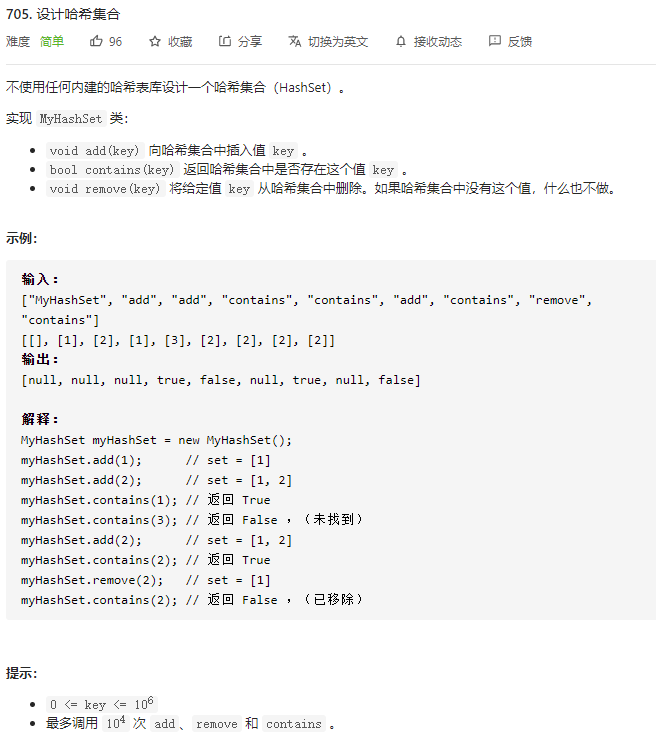思路一：朴实无华且枯燥的链地址法，通过 v e c t o r 、 f o r w a r d _ l i s t vector、forward\_list 实现(后者是一个单向链表)。

class MyHashSet {
public:
/** Initialize your data structure here. */
const int num=2333;
vector<forward_list<int>> hashTable;
MyHashSet() {
hashTable.resize(num);
}

if(!contains(key))
hashTable[key%num].push_front(key);
}

void remove(int key) {
int idx=key%num;
forward_list<int>::iterator it=hashTable[idx].before_begin();
forward_list<int>::iterator nxt=hashTable[idx].begin();
forward_list<int>::iterator ed=hashTable[idx].end();
while(nxt!=ed&&*nxt!=key)
it=nxt++;
if(nxt!=ed)
hashTable[idx].erase_after(it);
}

/** Returns true if this set contains the specified element */
bool contains(int key) {
int idx=key%num;
forward_list<int>::iterator it=hashTable[idx].begin();
forward_list<int>::iterator ed=hashTable[idx].end();
while(it!=ed&&*it!=key)
++it;
return it!=ed;
}
};

/**
* Your MyHashSet object will be instantiated and called as such:
* MyHashSet* obj = new MyHashSet();
* obj->remove(key);
* bool param_3 = obj->contains(key);
*/

思路二：位运算实现哈希，这个思想相当巧妙。考虑用一个 u n s i g n e d   l o n g   l o n g unsigned\ long\ long 数组实现哈希，不妨设模数为 b a s e base ，那么对于任意 k e y key ，我们可以把它转换为两部分： ⌊ k e y / b a s e ⌋ \lfloor key/base \rfloor k e y % b a s e key\%base ，第一部分决定它在数组中的下标，第二部分决定它在该处值的二进制表示的第几位(这里看代码应该更加直观)。考虑到 u l l ull 类型的取值范围为 [ 0 , 2 64 − 1 ] [0,2^{64}-1] ，一个 u l l ull 类型最多对应64个数值，所以应取 b a s e = 64 base=64 ，数组的范围应该为 ⌊ m a x _ k e y / b a s e ⌋ + 1 \lfloor max\_key/base \rfloor+1

class MyHashSet {
public:
/** Initialize your data structure here. */
const int base=64;
vector<unsigned long long> hashTable;
MyHashSet() {
int max=1e6,num=max/base;
if(num*base==max)
++num;
hashTable.resize(num);
}

hashTable[key/base]|=1ull<<(key%base);
}

void remove(int key) {
hashTable[key/base]&=~(1ull<<(key%base));
}

/** Returns true if this set contains the specified element */
bool contains(int key) {
return hashTable[key/base]&(1ull<<(key%base));
}
};

/**
* Your MyHashSet object will be instantiated and called as such:
* MyHashSet* obj = new MyHashSet();
* obj->remove(key);
* bool param_3 = obj->contains(key);
*/

展开全文• 为什么要使用哈希表 查找和插入是查找表的两项基本操作，对于单纯使用链表，数组，或二叉树实现的查找表来说，这两项操作在时间消耗上仍显得比较昂贵。 以查找为例：在数组实现的查找表中，需要用二分等查找方式...哈希表 哈希函数 哈希冲突 散列
• 一.哈希表 ...哈希函数的构造原则是：函数本身便于计算、计算出来的地址分布均匀（即对任意K，f(K)对应不同地址的概率相等）。 1.数字分析法： 可以从关键如果事先知道关键字集合，并且每个关键字的哈希算法 算法
• 哈希表定义 1.哈希表定义 哈希表是根据键值(Key value)而直接进行访问的数据结构。也就是说，它通过把关键码值映射到表中一个位置来访问记录，以加快查找的速度。这个映射函数叫做散列函数，存放记录的数组叫做散...数据结构
• 哈希算法

2021-01-15 14:36:07
哈希 1.什么是哈希？ 2.哈希函数？ 1.哈希函数的定义： 2.哈希函数的设计原理： 3.常见的哈希函数： 3.哈希冲突 1.闭散列： 2.开散列： 1.什么是哈希？ 按照某种方式，将元素与其所在的表格中的存储...
• 哈希和哈希桶

2021-10-31 23:54:34
概念 数据结构中有一类常用于搜索，给定一个元素集合，常见的可用于搜索的算法有 遍历，遍历是十分粗暴简单的手段，对于元素的集合没有特殊要求，时间复杂度是O(N)。...真实的效率决于比较的次数。 如哈希算法 数据结构 算法
• 然后通过哈希算法（如余法等）获得的值比方0，就是哈希值（位置信息），存储“0对应老铁双击666“这些关系的就是哈希表。 什么是哈希冲突？ 图1 上面的30比方就是老铁双击666，但为什么后面还有值呢，因为其他值...哈希 哈希算法
• 哈希表！！

2021-08-24 17:04:13
H(key) 和处理冲突的方法将一组关键字映像到一个有限的连续的地址集(区间)上，并以关键字在地址集中的“像”作为记录在表中的存储位置，这种表便称为哈希表，这一映像称为哈希造表或散列，所得存储位置称哈希地址或...哈希表 散列表 数据结构
• 以数据中每个元素的关键字K为自变量，通过散列函数H（k）计算出函数值，以该函数值作为一块连续存储空间的的单元地址，将该元素存储到函数值对应的单元中。 3、哈希表查找的时间复杂度 哈希表...数据结构 哈希表
• 该楼层疑似违规已被系统折叠隐藏此楼查看此楼}// ***********************显示哈希表***********************void DisplayHashTable(){int i;printf("\n\n 地址 \t\t 姓名 \t\t 关键字\n");for (i=0;i{printf("%2d %...
• 哈希算法（哈希函数）基本

千次阅读 2021-09-19 16:33:42
一、什么是哈希（Hash） 哈希也称“散列”函数或“杂凑”函数。它是一个不可逆的单向映射，将任意长度的输入消息M（或文件F）映射成为一个较短的定长哈希值H（M），也叫散列值（HashValue）、杂凑值或消息摘要。...算法
• 为了解决哈希冲突，我们一般应用开放定址法、链地址法、建立公共溢出区、再哈希法四种方式 方法一：链地址法 这里为了节约空间，采用链地址法 注意：list不是连续内存,list 容器底层是用双向链表实现的,因此遍历...
• 一致性哈希

2021-01-30 15:51:38
那么我们要说的一致性哈希算法，其实就是解决了这里面的 存取规则 的问题，有了这个一致性哈希算法，我们能够准确的知道我们要的数据落在哪个机器的哪个数据库中。 1. 简单哈希 还是上面水平拆分数据库的例子,假设...
• 哈希表介绍

2021-05-25 21:00:02
随机数法：选择一个随机函数，关键字的随机函数值为它的哈希地址，即 H(key) = random(key)，其中 random 为随机数函数，通常关键字长度不等时采用此法； 数学分析法：设有 n 个 d 位数，每一位可能有 r 种不同的...数据结构 哈希表 闭散列
• function HashTable(){ this.storage=[]; this.count=0//记录哈希表中已经存放了多少元素,决定数组是扩容还是减少容量 this.limit=7;... /* 函数使index在数组长度内index比较均匀， 1.在哈希表中
• C语言哈希表

2021-11-21 10:01:06
1.概念 哈希表：关键字与存储位置有函数关系的表。...随机关键字在哈希表上分布的越均匀，发生冲突的概率也越低，这是构造哈希表要遵循的原则，还有计算的函数要尽量简单，不然会拖慢运行速度。 设关键字为key，H(kc语言 数据结构 哈希表
• 哈希表

2021-03-30 22:49:45
顺序结构以及平衡树中，元素关键码与其存储位置之间没有对应的关系，因此在查找一个元素时，必须要经过关键码的多次比较，顺序查找时间复杂度为O(N)，平衡树中为树的高度，即O(logN)，搜索的效率决于搜索过程中...数据结构 java 哈希表
• 文章目录哈希哈希（散列）函数 哈希 哈希（散列）是一种数据结构，通过散列算法将元素值转换为散列值进行存储。使得元素存储的位置与元素...哈希函数计算出来的地址能均匀分布在整个空间中（防止产生密集的哈希冲突数据结构 算法
• 顺序结构以及平衡树中，元素关键码与其存储位置之间没有对应关系，因此在查找一个元素的时候，必须要经过关键码的多次比较，这样查找的效率就比较低下，搜索的效率决于搜索过程中元素的比较次数。 理想的搜索...
• 解决哈希冲突

2021-11-25 17:08:40
哈希法又称散列法、杂凑法以及关键字地址计算法等，相应的表成为哈希表。 基本思想：首先在元素的关键字K和元素的位置P之间建立一个对应关系f，使得P=f(K),其中f成为哈希函数。 创建哈希表时，把关键字K的元素直接...java 开发语言
•     最近在刷题以及做编程练习的作业时经常会用到哈希表，碰到一些想用的函数时每次都看别人的博客，现结合别人的博客对哈希表做个总结。 本篇哈希表的作用如何使用STL库中的哈希表STL中哈希表的常用函数 哈希表...c++ 哈希表
• 数据结构——哈希哈希表介绍 1、什么是哈希表？ 可以考虑这么一个情景： 现在某个学校为新生录入信息，新生们的学号为2001、2002、2003…这样的类型，现将其存储在一个数组中，现在要查找学号为2066的学生。 ...java 数据结构 散列表 哈希算法 c++
• C++ 哈希表

千次阅读 2021-11-22 21:07:08
什么是哈希表 map、hash_map、unordered_map的引入 unordered_map的用法 1. 什么是哈希表 1.1 哈希表的定义 “散列表（Hash table，也叫哈希表），是根据键（Key）而直接访问在内存存储位置的数据结构。也就是说，...linux 运维 服务器
• 一、定义 哈希表（Hash table，也叫散列表），是根据关键码值(Key value)而直接进行访问的数据结构。...关键字被某个不大于哈希表长m的数p除后所得的余数为哈希地址。即： H(key)=key MODE p,p<=m....